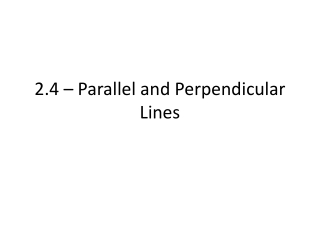DownloadDownload Presentation2.4 – Parallel and Perpendicular Lines

# 2.4 – Parallel and Perpendicular Lines

Download Presentation## 2.4 – Parallel and Perpendicular Lines

- - - - - - - - - - - - - - - - - - - - - - - - - - - E N D - - - - - - - - - - - - - - - - - - - - - - - - - - -
##### Presentation Transcript

1. 2.4 – Parallel and Perpendicular Lines

2. Recall… • Slope = measure of steepness of a particular line/graph of an equation • m = Rise/Run OR

3. We can also look at slopes of multiple lines • Parallel ( || ): two lines are considered parallel, if and only if, their rise and run are both the same amount • IE, m1 = m2 • Never intersect

4. Example. Find an equation, in slope-intercept form, that is parallel to the line 3x + 2y = 3y – 7 and passes through (1,2). • How do we find the slope of the given line?

5. Perpendicular ( ): two lines are perpendicular, if and only if, they are opposite reciprocals OR m1(m2) = -1 • If horizontal and vertical, slopes of undefined and zero must occur • Intersection is 900 angle

6. Example. Find an equation, in slope-intercept form, that is perpendicular to the line 3x + y = 7 and passes through the point (-2, 5). • How can we find the given slope? • Same? OR?

7. Example. Determine if the two lines 3x + y = 2 and x + 3y = 2 are perpendicular, parallel, or neither.

8. Example. A parallelogram is a quadrilateral formed by two pairs of opposite parallel sides. Determine if following points form a quadrilateral (vertices listed counter-clockwise). • {(-2,2), (-5,-2), (2, -3), (5,1)}

9. Assignment • Pg. 161 • 1-25 even, 33